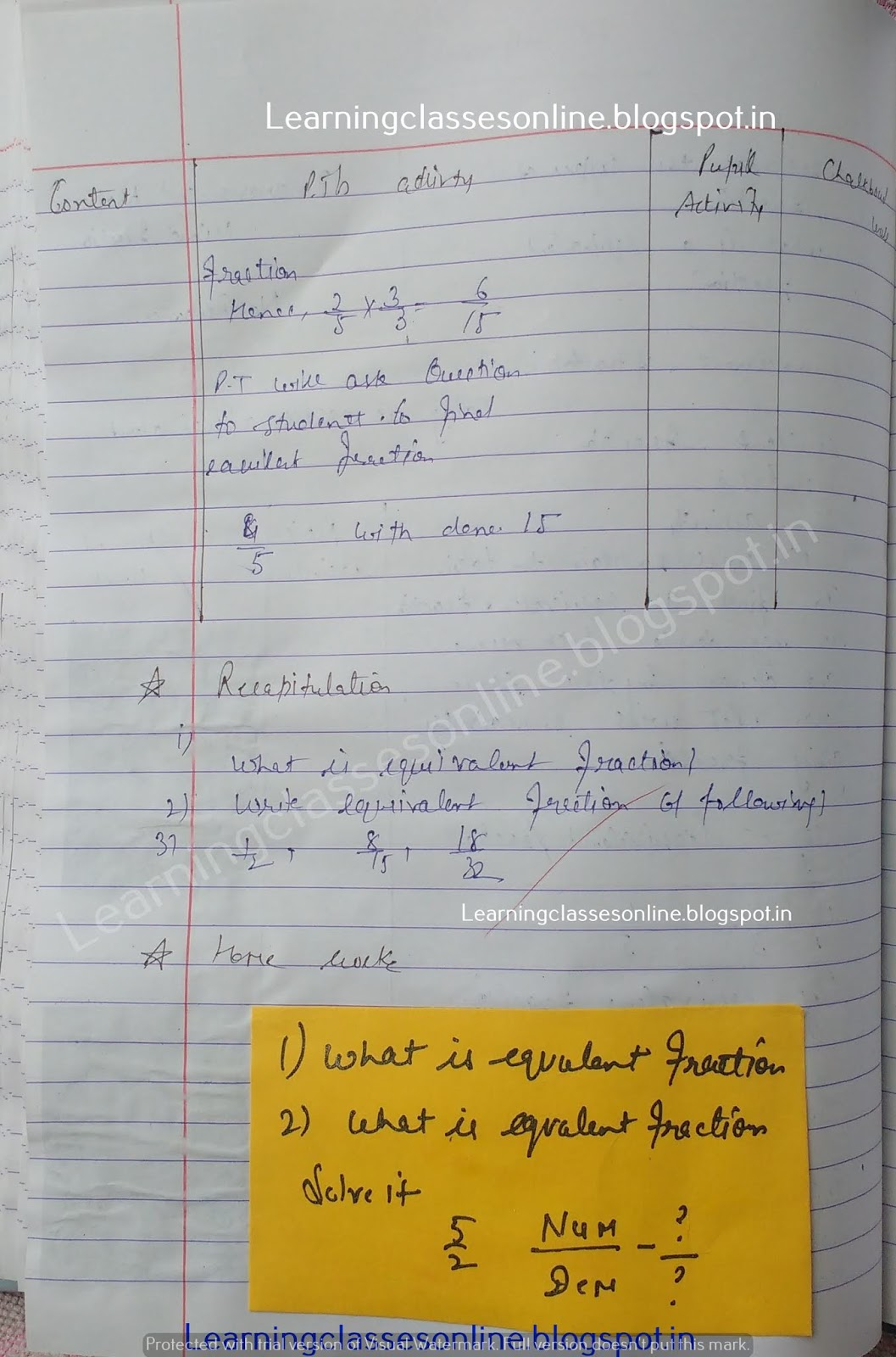Math Lesson Plan on Equivalent Fraction

# Math Lesson Plan on Equivalent Fraction

Math Lesson Plan on Equivalent Fraction  for grade 6 , 7 and 8

Note: The Mathematics Lesson Plan given below is just an example. You can change the Name, Class, Course, Date, Duration, etc. according to your needs.Further Reference:
www.learningclassesonline.com Mathematics Lesson Plan for B.Ed